# Texas Go Math Grade 3 Lesson 3.5 Answer Key Equivalent Fractions

Refer to our Texas Go Math Grade 3 Answer Key Pdf to score good marks in the exams. Test yourself by practicing the problems from Texas Go Math Grade 3 Lesson 3.5 Answer Key Equivalent Fractions.

## Texas Go Math Grade 3 Lesson 3.5 Answer Key Equivalent Fractions

Essential Question
How can you use models to name equivalent fractions?
Equivalent fractions are different fractions that name the same number.
Examples:
Two-thirds is equivalent to four-sixths. The fractions three-fourths, six-eighths, and nine-twelfths are equivalent.

Unlock the problem
Cole brought a submarine sandwich to the picnic. He shared the sandwich equally with 3 friends. The sandwich was cut into eighths. What are two ways to describe the part of the sandwich each friend ate?

• How many people shared the sandwich?
It is given that
Cole brought a submarine sandwich to the picnic. He shared the sandwich equally with 3 friends. The sandwich was cut into eighths
Hence, from the above,
We can conclude that
The number of people that shared the sandwich is: 3 people

Cole grouped the smaller pieces into twos. Draw circles to show equal groups of two pieces to show what each friend ate.There are 4 equal groups. Each group is $$\frac{1}{4}$$ of the whole sandwich. So, each friend ate $$\frac{1}{4}$$ of the whole sandwich.
How many eighths did each friend eat?
The number of eighths did each friend eat is: 2 eighths

$$\frac{1}{4}$$ and $$\frac{2}{8}$$ are equivalent fractions since they both name the same amount of the sandwich.
So,
$$\frac{1}{4}$$ and $$\frac{2}{8}$$ of the sandwich are two ways to describe the part of the sandwich each friend ate.

Example Model the problem.
Heidi ate $$\frac{3}{6}$$ of her fruit bar. Molly ate $$\frac{4}{8}$$ of her fruit bar, which is the same size. Which girl ate more of her fruit bar?
Shade $$\frac{3}{6}$$ of Heidi’s fruit bar and $$\frac{4}{8}$$ of Molly’s fruit bar.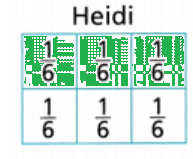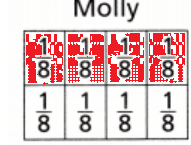• Is $$\frac{3}{6}$$ greater than, less than, or equal to $$\frac{4}{8}$$?
The given fractions are:
$$\frac{3}{6}$$ and $$\frac{4}{8}$$
So,
$$\frac{3}{6}$$ = $$\frac{4}{8}$$ = $$\frac{1}{2}$$
Hence, from the above,
We can conclude that
$$\frac{3}{6}$$ is equal to $$\frac{4}{8}$$
So,
Both girls ate the same amount.

Share and Show

Question 1.
Each shape is 1 whole. Use the model to find the equivalent fraction.The given model is:Now,
From the above model,
We can observe that
The model with $$\frac{1}{2}$$ is divided into further 2 parts. So,
The total parts are: 4
So,
The equivalent fraction of $$\frac{1}{2}$$ is: $$\frac{2}{4}$$
Hence, from the above,
We can conclude that
The equivalent fraction of $$\frac{1}{2}$$ is: $$\frac{2}{4}$$

Math Talk
Mathematical Processes
Explain why both fractions name the same amount?
The models from the above problem are:Now,
From the above models,
We can observe that
$$\frac{1}{2}$$ and $$\frac{1}{4}$$ have the same model since the model of $$\frac{1}{2}$$ is further divided
Hence, from the above,
We can conclude that
Both fractions name the same amount

Each shape is 1 whole. Shade the model or use the number line to find the equivalent fraction.

Question 2.The given model is: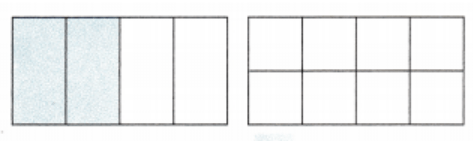Now,
The representation of the equivalent fraction in the given model is:Hence, from the above,
We can conclude that
$$\frac{2}{4}$$ = $$\frac{4}{8}$$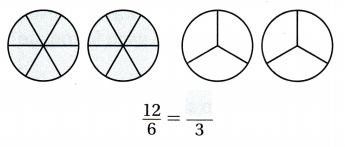The given model is: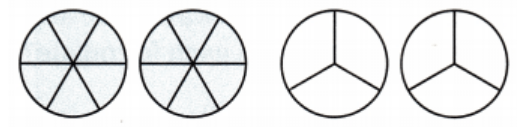Now,
The representation of the equivalent fraction in the given model is: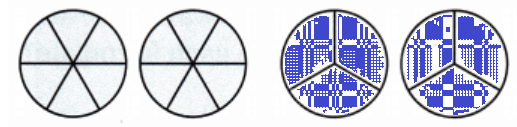Hence, from the above,
We can conclude that
$$\frac{12}{6}$$ = $$\frac{2}{3}$$

Question 4.
Andy swam $$\frac{8}{8}$$ mile in a race. Use the number line to find a fraction that is equivalent to $$\frac{8}{8}$$.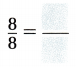It is given that
Andy swam $$\frac{8}{8}$$ mile in a race
Now,
The given number line is:Now,
From the above number line,
We can observe that
$$\frac{8}{8}$$ = $$\frac{4}{4}$$
Hence, from the above,
We can conclude that
$$\frac{8}{8}$$ = $$\frac{4}{4}$$

Circle equal groups to find the equivalent fraction.

Question 5.The given model is: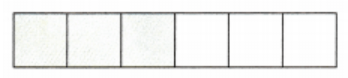Now,
The representation of the given model in the form of groups to represent the equivalent fraction is:Hence, from the above,
We can conclude that
$$\frac{3}{6}$$ = $$\frac{1}{2}$$

Question 6.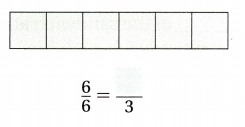The given model is: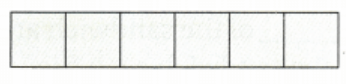Now,
The representation of the given model in the form of groups to represent the equivalent fraction is:Hence, from the above,
We can conclude that
$$\frac{6}{6}$$ = $$\frac{3}{3}$$

Write the fraction that names the shaded part of each circle.Which pairs of fractions are equivalent?
The given models are: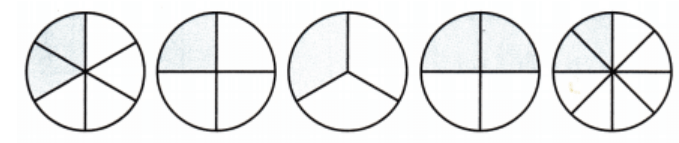Now,
From the above models,
The representations of the fractions from the first model to the last model respectively are:
$$\frac{2}{6}$$, $$\frac{1}{4}$$, $$\frac{1}{3}$$, $$\frac{2}{4}$$, $$\frac{2}{8}$$
Hence, from the above,
We can conclude that
The pairs of fractions that are equivalent is:
$$\frac{2}{6}$$ = $$\frac{1}{3}$$
$$\frac{1}{4}$$ = $$\frac{2}{8}$$

Problem Solving

Question 8.
H.O.T. Write Math After dessert, $$\frac{2}{3}$$ of a cherry pie is left. Suppose 4 friends want to share it equally. What fraction names how much of the whole pie each friend will get? Use the model on the right.It is given that
After dessert, $$\frac{2}{3}$$ of a cherry pie is left. Suppose 4 friends want to share it equally.
Now,
The given model is:Now,
From the given information,
The fraction of the pie each friend will get = (The number of friends) × (The total fraction of the pie)
= 4 × $$\frac{2}{3}$$
= $$\frac{8}{3}$$
Hence, from the above,
We can conclude that
The fraction of the pie each friend will get is: $$\frac{8}{3}$$ of the pie

Question 9.
Apply There are 16 people having lunch. Each person wants $$\frac{1}{4}$$ of a pizza. How many whole pizzas are needed? Draw a picture to show your answer.
It is given that
There are 16 people having lunch. Each person wants $$\frac{1}{4}$$ of a pizza
Now,
According to the given information,
The number of pizzas that are needed = (The total number of people having lunch) × (The fraction of the pizza each friend wants)
= 16 × $$\frac{1}{4}$$
= 4 pizzas
Hence, from the above,
We can conclude that
The number of pizzas that are needed is: 4 pizzas

H.O.T. Lucy has 5 brownies. Each brownie is cut in half. What fraction names all of the brownie halves??
It is given that
Lucy has 5 brownies. Each brownie is cut in half
Now,
According to the given information,
The number of brownie halves = (The number of brownies Lucy has) × (The number f pieces the brownie is cut into)
= 5 × $$\frac{1}{2}$$
= $$\frac{5}{2}$$ brownie halves

What if Lucy cuts each part of the brownie into 2 equal pieces to share with friends? What fraction names all of the brownie pieces now?are equivalent fractions.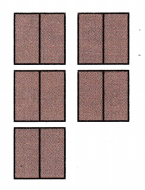It is given that
Lucy cuts each part of the brownie into 2 equal pieces to share with friends
Now,
The given model is:Now,
According to the given information,
The fraction of all the pieces of brownie Lucy has = (The total number of brownies) ÷ (The number of groups of brownies)
= $$\frac{10}{4}$$
Hence, from the above,
We can conclude that
$$\frac{5}{2}$$ = $$\frac{10}{4}$$

Question 11.
Multi-Step Christy bought 8 muffins. She chose 2 chocolate, 2 banana, and 4 blueberries. She and her family ate the chocolate and banana muffins for breakfast. What fraction of the muffins did they eat? Write an equivalent fraction. Draw a picture.
It is given that
Christy bought 8 muffins. She chose 2 chocolate, 2 banana, and 4 blueberries. She and her family ate the chocolate and banana muffins for breakfast
Now,
According to the given information,
The fraction of the muffins did Christy and her family ate = (The number of muffins Christy and her family ate) ÷ (The total number of muffins Christy bought)
= $$\frac{4}{8}$$
= $$\frac{1}{2}$$
Hence, from the above,
We can conclude that
The fraction of the muffins did Christy and her family ate is: $$\frac{4}{8}$$ of the muffins
The equivalent fraction of the muffins did Christy and her family ate is: $$\frac{1}{2}$$

Fill in the bubble for the correct answer choice.

Question 12.
A team of scientists cut a slice of a tree into six equal pieces to determine its age. There are three scientists, so each gets $$\frac{1}{3}$$ of the slice. Which fraction is equivalent to $$\frac{1}{3}$$?
(A) $$\frac{1}{6}$$
(B) $$\frac{2}{6}$$
(C) $$\frac{3}{6}$$
(D) $$\frac{4}{6}$$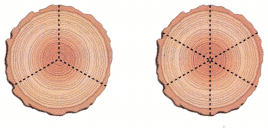It is given that
A team of scientists cut a slice of a tree into six equal pieces to determine its age. There are three scientists, so each gets $$\frac{1}{3}$$ of the slice
Now,
The given model is:Now,
From the above model,
We can observe that
$$\frac{1}{3}$$ = $$\frac{2}{6}$$
Hence, from the baove,
We can conclude that
The fraction that is equivalent to $$\frac{1}{3}$$ is: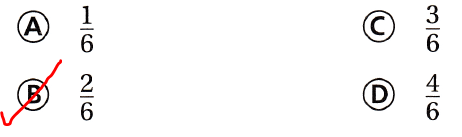Question 13.
Simone ate $$\frac{3}{6}$$ of her sandwich. Which fraction is equivalent to $$\frac{3}{6}$$?
(A) $$\frac{1}{4}$$
(B) $$\frac{1}{3}$$
(C) $$\frac{1}{2}$$
(D) $$\frac{2}{3}$$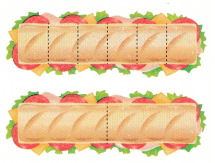It is given that
Simone ate $$\frac{3}{6}$$ of her sandwich
Now,
According to the given information,
The fraction that is equal to $$\frac{3}{6}$$ = $$\frac{1}{2}$$
Hence, from the above,
We can conclude that
The fraction that is equivalent to $$\frac{3}{6}$$ is:Question 14.
Representations Multi-Step Sally ate $$\frac{2}{8}$$ of her tortilla. Dylan ate $$\frac{2}{3}$$ of his tortilla. Michael ate $$\frac{1}{2}$$ of his tortilla. Bianca ate $$\frac{1}{4}$$ of her tortilla. Which friends had the same fraction of their tortillas left?
(A) Dylan and Michael
(B) Sally and Dylan
(C) Dylan and Bianca
(D) Sally and Bianca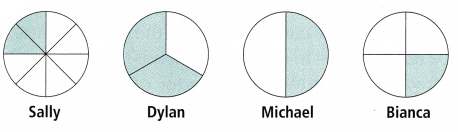It is given that
Sally ate $$\frac{2}{8}$$ of her tortilla. Dylan ate $$\frac{2}{3}$$ of his tortilla. Michael ate $$\frac{1}{2}$$ of his tortilla. Bianca ate $$\frac{1}{4}$$ of her tortilla
Now,
The given model is:Now,
Let the whole part of the torill be: 1
Now,
From the above model,
We can observe that
The fraction of the torilla left by Sally = 1 – $$\frac{2}{8}$$
= $$\frac{6}{8}$$
The fraction of the torilla left by Dylan = 1 – $$\frac{2}{3}$$
= $$\frac{1}{3}$$
The fraction of the torilla left by Michael = 1 – $$\frac{1}{2}$$
= $$\frac{1}{2}$$
The fraction of the torilla left by Bianca = 1 – $$\frac{1}{4}$$
= $$\frac{3}{4}$$
Now,
From the above fractions,
We can observe that
$$\frac{3}{4}$$ = $$\frac{6}{8}$$
Hence, from the above,
We can conclude that
The friends that had the same fraction of their tortillas left is:Texas Test Prep

Question 15.
Mr. Peters made an apple pie. There is $$\frac{6}{8}$$ of the pie leftover. Which fraction is equal to the part of the pie that is left over?
(A) $$\frac{3}{4}$$
(B) $$\frac{2}{4}$$
(C) $$\frac{1}{2}$$
(D) $$\frac{1}{4}$$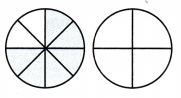It is given that
Mr. Peters made an apple pie. There is $$\frac{6}{8}$$ of the pie leftover
So,
According to the given information,
The equivalent fraction of the pie that is leftover is: $$Hence, from the above, We can conclude that The equivalent fraction of the pie that is leftover is:### Texas Go Math Grade 3 Lesson 3.5 Homework and Practice Answer Key Each shape is 1 whole. Shade the model to find the equivalent fraction. Question 1.Answer: The given model is: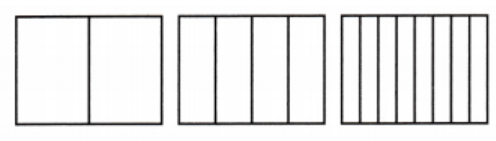Now, The representation of the given model with the equivalent fractions is: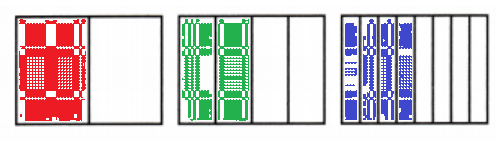Hence, from the above, We can conclude that [latex]\frac{1}{2}$$ = $$\frac{2}{4}$$ = $$\frac{4}{8}$$The given model is:Now,
The representation of the given model with the equivalent fractions is: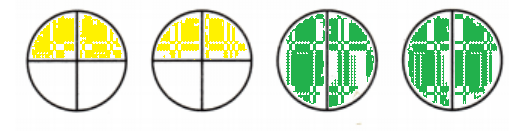Hence, from the above,
We can conclude that
$$\frac{8}{4}$$ = $$\frac{4}{2}$$

Question 3.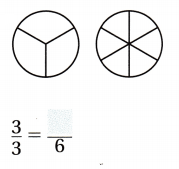The given model is:Now,
The representation of the given model with the equivalent fractions is: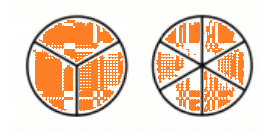Hence, from the above,
We can conclude that
$$\frac{3}{3}$$ = $$\frac{6}{6}$$

Question 4.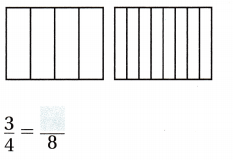The given model is: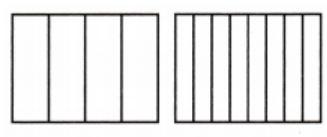Now,
The representation of the given model with the equivalent fractions is:Hence, from the above,
We can conclude that
$$\frac{3}{4}$$ = $$\frac{6}{8}$$

Problem Solving

Question 5.
Tom ate $$\frac{2}{6}$$ of his granola bar. Hank ate $$\frac{1}{3}$$ of his granola bar.
Shade $$\frac{2}{6}$$ of Tom’s granola bar and $$\frac{1}{3}$$ of Hank’s granola bar. Who ate more of the granola bar?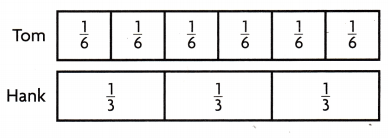It is given that
Tom ate $$\frac{2}{6}$$ of his granola bar. Hank ate $$\frac{1}{3}$$ of his granola bar.
Shade $$\frac{2}{6}$$ of Tom’s granola bar and $$\frac{1}{3}$$ of Hank’s granola bar.
Now,
The representation of models that represent the eating of a granola bar is: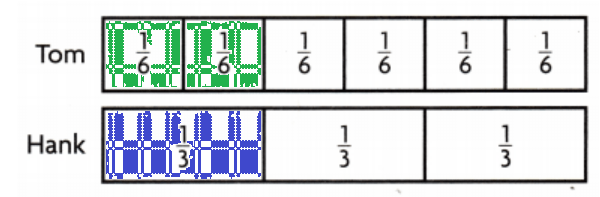So,
$$\frac{2}{6}$$ = $$\frac{1}{3}$$
Hence, from the above,
We can conclude that
Tom and Hank ate the same amount of granola bar

Lesson Check

Matt cut his mini pizza into six equal pieces and ate two of them. Dawn cut her mini pizza into three equal pieces and ate one piece.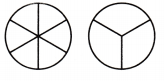Which statement about the amount each ate is true?
(A) Mati ate $$\frac{2}{3}$$ pizza and Dawn ate $$\frac{1}{3}$$ pizza. $$\frac{2}{3}$$ = $$\frac{1}{3}$$
(B) Matt ate $$\frac{2}{6}$$ pizza and Dawn ate $$\frac{1}{3}$$ pizza. $$\frac{2}{6}$$ > $$\frac{1}{3}$$
(C) Mati ate $$\frac{2}{6}$$ pizza and Dawn ate $$\frac{1}{3}$$ pizza. $$\frac{2}{6}$$ < $$\frac{1}{3}$$
(D) Matt ate pizza and Dawn ate $$\frac{1}{3}$$ pizza. $$\frac{2}{6}$$ = $$\frac{1}{3}$$
It is given that
Matt cut his mini pizza into six equal pieces and ate two of them. Dawn cut her mini pizza into three equal pieces and ate one piece.
Now,
The representation of the models that Matt and dawn ate respectively is:So,
From the above models,
We can say that
$$\frac{2}{6}$$ = $$\frac{1}{3}$$
Hence, from the above,
We can conclude that
The statement that is true about the amount of pizza both ate is:Question 7.
Beth and Bonnie bought a submarine sandwich. Beth cut the sandwich into four equal parts. Each girl ate half of her sandwich.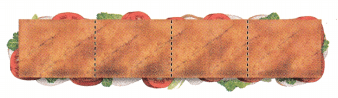Which are equivalent fractions that show how much of the sandwich each girl ate?
(A) $$\frac{1}{4}$$ = $$\frac{1}{2}$$
(B) $$\frac{2}{4}$$ = $$\frac{1}{2}$$
(C) $$\frac{3}{4}$$ = $$\frac{1}{2}$$
(D) $$\frac{4}{4}$$ = $$\frac{2}{2}$$
It is given that
Beth and Bonnie bought a submarine sandwich. Beth cut the sandwich into four equal parts. Each girl ate half of her sandwich.
Now,
According to the given information,
The amount of the sandwich each girl ate is: $$\frac{1}{2}$$
Hence, from the above,
We can conclude that
The equivalent fractions that show how much of the sandwich each girl ate is: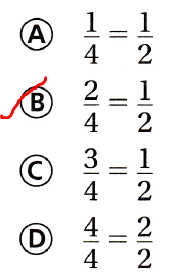Multi-Step The shaded models show the fractional part of a turkey sandwich that each person ate. Which people ate the same amount?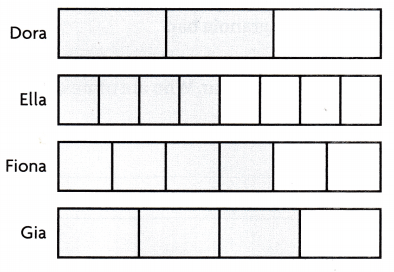(A) Ella and Gia
(B) Dora and Ella
(C) Dora and Fiona
(D) Fiona and Gia
It is given that
The shaded models show the fractional part of a turkey sandwich that each person ate.
Now,
The given models are:Now,
From the above,
We can observe that
The representation of the fractions of the given models is:
Dora – $$\frac{2}{3}$$
Ella – $$\frac{4}{8}$$
Fiona – $$\frac{4}{6}$$
Gia – $$\frac{3}{4}$$
Hence, from the above,
We can conclude that
The people who ate the same amount were:Scroll to Top